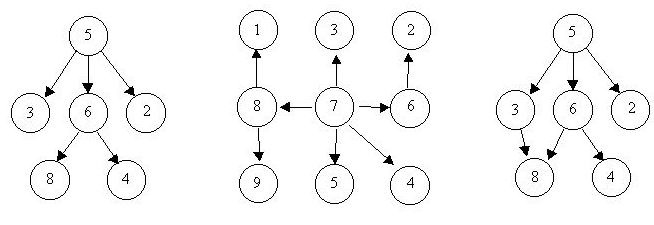Is It A Tree?

 Time Limit: 1000MS Memory Limit: 10000K Total Submissions: 32052 Accepted: 10876

Description

<div class=”ptx” lang=”en-US” style=”font-family:”Times New Roman”,Times,serif; font-size:14px”>
A tree is a well-known data structure that is either empty (null, void, nothing) or is a set of one or more nodes connected by directed edges between nodes satisfying the following properties.

There is exactly one node, called the root, to which no directed edges point.

Every node except the root has exactly one edge pointing to it.

There is a unique sequence of directed edges from the root to each node.

For example, consider the illustrations below, in which nodes are represented by circles and edges are represented by lines with arrowheads. The first two of these are trees, but the last is not.In this problem you will be given several descriptions of collections of nodes connected by directed edges. For each of these you are to determine if the collection satisfies the definition of a tree or not.

Input

<div class=”ptx” lang=”en-US” style=”font-family:”Times New Roman”,Times,serif; font-size:14px”>
The input will consist of a sequence of descriptions (test cases) followed by a pair of negative integers. Each test case will consist of a sequence of edge descriptions followed by a pair of zeroes Each edge description will consist of a pair of integers;
the first integer identifies the node from which the edge begins, and the second integer identifies the node to which the edge is directed. Node numbers will always be greater than zero.

Output

<div class=”ptx” lang=”en-US” style=”font-family:”Times New Roman”,Times,serif; font-size:14px”>
For each test case display the line “Case k is a tree.” or the line “Case k is not a tree.”, where k corresponds to the test case number (they are sequentially numbered starting with 1).

Sample Input

```6 8  5 3  5 2  6 4
5 6  0 0

8 1  7 3  6 2  8 9  7 5
7 4  7 8  7 6  0 0

3 8  6 8  6 4
5 3  5 6  5 2  0 0
-1 -1```

Sample Output

```Case 1 is a tree.
Case 2 is a tree.
Case 3 is not a tree.```

Source

North Central North America 1997

```#include
#include
#include

const int maxn = 1e6+7;

using namespace std;

int father[maxn], n;

void init()
{
for (int i=0; i<=maxn; i++) father[i]="i;" } int fi(int x) { return x="=" father[x] ? : void unite(int x, y) p1="fi(x)," p2="fi(y);" if (p1="=" p2) return; father[p1]="p2;" same(int 1; 0; main() a, b; c="1;" vector v;
init();bool judge = true;
while (~scanf("%d%d", &a, &b))
{
if (a == -1 && b == -1) break;
if (a == 0 && b == 0) {
for (int i=0; i
```

• 本文作者： Fayne
• 本文链接： 347.html
• 版权声明： 本博客所有文章除特别声明外，均采用 CC BY-NC-SA 4.0 许可协议。转载请注明出处！